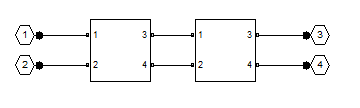Description

Use the cascade object to represent cascaded networks of RF objects that are characterized by the components that make up the individual network. The following figure shows the configuration of a pair of cascaded networks.Creation

Description

h = rfckt.cascade returns a cascaded network object whose properties all have their default values.

example

h = rfckt.cascade('Ckts',value) returns a cascaded network with elements specified in the name-value pair property Ckts.

Properties

expand all

Computed S-parameters, noise figure, OIP3, and group delay values. For more information, see Algorithms.

Data Types: function_handle

Circuit objects in network. All circuits must be 2-port. By default, this property is empty.

Data Types: char

Data Types: char

Number of ports of cascaded network. The default value is 2.

Data Types: double

Object Functions

 analyze Analyze RFCKT object in frequency domain calculate Calculate specified parameters for rfckt objects or rfdata objects circle Draw circles on Smith Chart extract Extract specified network parameters from rfckt object or data object listformat List valid formats for specified circuit object parameter listparam List valid parameters for specified circuit object loglog Plot specified circuit object parameters using log-log scale plot Plot circuit object parameters on X-Y plane plotyy Plot parameters of RF circuit or RF data on X-Y plane with two Y-axes getop Display operating conditions polar Plot specified object parameters on polar coordinates semilogx Plot RF circuit object parameters using log scale for x-axis semilogy Plot RF circuit object parameters using log scale for y-axis smith Plot circuit object parameters on Smith chart write Write RF data from circuit or data object to file getz0 Calculate characteristic impedance of RFCKT transmission line object read Read RF data from file to new or existing circuit or data object restore Restore data to original frequencies getop Display operating conditions groupdelay Group delay of S-parameter object or RF filter object or RF Toolbox circuit object

Examples

collapse all

Create a cascade network using rfckt.cascade with amplifier and transmission lines as elements.

amp = rfckt.amplifier('IntpType','cubic');
tx1 = rfckt.txline;
tx2 = rfckt.txline;
casccircuit =

Ckts: {1x3 cell}
nPort: 2
AnalyzedResult: []

Algorithms

The analyze method computes the AnalyzedResult property using the data stored in the Ckts property as follows:

• The analyze method starts calculating the ABCD-parameters of the cascaded network by converting each component network's parameters to an ABCD-parameters matrix. The figure shows a cascaded network consisting of two 2-port networks, each represented by its ABCD matrix.

The analyze method then calculates the ABCD-parameters matrix for the cascaded network by calculating the product of the ABCD matrices of the individual networks.

The following figure shows a cascaded network consisting of two 2-port networks, each represented by its ABCD-parameters.The following equation illustrates calculations of the ABCD-parameters for two 2-port networks.

$\left[\begin{array}{cc}A& B\\ C& D\end{array}\right]=\left[\begin{array}{cc}A\prime & B\prime \\ C\prime & D\prime \end{array}\right]\left[\begin{array}{cc}A\prime \prime & B\prime \prime \\ C\prime \prime & D\prime \prime \end{array}\right]$

Finally, analyze converts the ABCD-parameters of the cascaded network to S-parameters at the frequencies specified in the analyze input argument freq.

• The analyze method calculates the noise figure for an N-element cascade. First, the method calculates noise correlation matrices CA' and CA, corresponding to the first two matrices in the cascade, using the following equation:

${C}_{A}=2kT\left[\begin{array}{cc}{R}_{n}& \frac{N{F}_{\mathrm{min}}-1}{2}-{R}_{n}{Y}_{opt}{}^{\ast }\\ \frac{N{F}_{\mathrm{min}}-1}{2}-{R}_{n}{Y}_{opt}& {R}_{n}{|{Y}_{opt}|}^{2}\end{array}\right]$

where k is Boltzmann's constant, and T is the noise temperature in Kelvin.

The method combines CA' and CA into a single correlation matrix CA using the equation

${C}_{A}={C}_{A}^{\text{'}}+\left[\begin{array}{cc}A\text{'}& B\text{'}\\ C\text{'}& D\text{'}\end{array}\right]{C}_{A}^{\text{'}\text{'}}\left[\begin{array}{cc}A\text{'}& B\text{'}\\ C\text{'}& D\text{'}\end{array}\right]$

By applying this equation recursively, the method obtains a noise correlation matrix for the entire cascade. The method then calculates the noise factor, F, from the noise correlation matrix of as follows:

$\begin{array}{l}F=1+\frac{{z}^{+}{C}_{A}z}{2kT\mathrm{Re}\left\{{Z}_{S}\right\}}\\ z=\left[\begin{array}{c}1\\ {Z}_{S}{}^{*}\end{array}\right]\end{array}$

In the two preceding equations, ZS is the nominal impedance, which is 50 ohms, and z+ is the Hermitian conjugation of z.

• The analyze method calculates the output power at the third-order intercept point (OIP3) for an N-element cascade using the following equation:

$OI{P}_{3}=\frac{1}{\frac{1}{OI{P}_{3,N}}+\frac{1}{{G}_{N}\cdot OI{P}_{3,N-1}}+\dots +\frac{1}{{G}_{N}\cdot {G}_{N-1}\cdot \dots \cdot {G}_{2}\cdot OI{P}_{3,1}}}$

where Gn is the gain of the nth element of the cascade and OIP3,N is the OIP3 of the nth element.

• The analyze method uses the cascaded S-parameters to calculate the group delay values at the frequencies specified in the analyze input argument freq, as described in the analyze reference page.

 Ludwig, R. and P. Bretchko, RF Circuit Design: Theory and Applications, Prentice Hall, 2000.